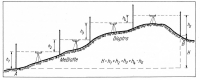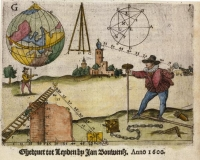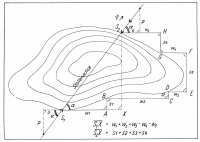Measure: Number or quantity that records a directly observable value or performance. All measures have a unit attached to them: inch, centimeter, liter, meter, etc. In contrast, an indicator is an indirect measure or a predictor (such as a leading Indicator) of performance.
Home Aqua Clopedia FAQ on aqueducts

Measures

Ancient sources give two quite different figures for a minimal acceptable gradient, and these are not uncommonly at odds with the gradient of a number of aqueducts. Vitruvius suggests 0.5% and Pliny specifies 0.02%. The aqueducts themselves range between 0.3% and 0.15%, with extremes of 0.07% at Nîmes and over 30% on other places.

The length of the aqueducts was expressed in passus ('steps'), a common Roman measurement. A passus corresponded to 1.482 m or 4 ft 10 1/4 inch. The next order of magnitude was the milia passus, or Roman mile. This actually meant 'thousands of steps'. A milia passus equals 1.482 km, or 0.92 mile.

The Romans also used the pes, or foot, a measurement they inherited. The pes varied through time from 0.2957 m to 0.2973 m. The 1/12 part of a pes was called uncia, 1/16 was called digitus and the 1/4 part palma. Five pedes made a passus, the standard double step of a soldier. To measure land surveyors used the actus, equal to 120 pedes or 24 passus. Two square actus made an iugerum (Hauck, 1988:36).

The rate of flow of each aqueduct was calculated in quinariae. It is an impossible task to determine exactly what a quinaria was, but scholars have calculated that one quinaria was something equal to 0.48 liters/second. The most powerful of the eleven aqueducts, the Anio Novus, drew 4,738 quinariae - according to Frontinus - which meant a supply of almost 200 million liters per day (There are 86,400 seconds in a day. A rate of 4,738 quinariae equals 2274.24 liters per second. The product of 2274.24 and 86,400 is 196,494,336).

From the thesis of Evan J. Dembskey on The aqueducts of Ancient Rome